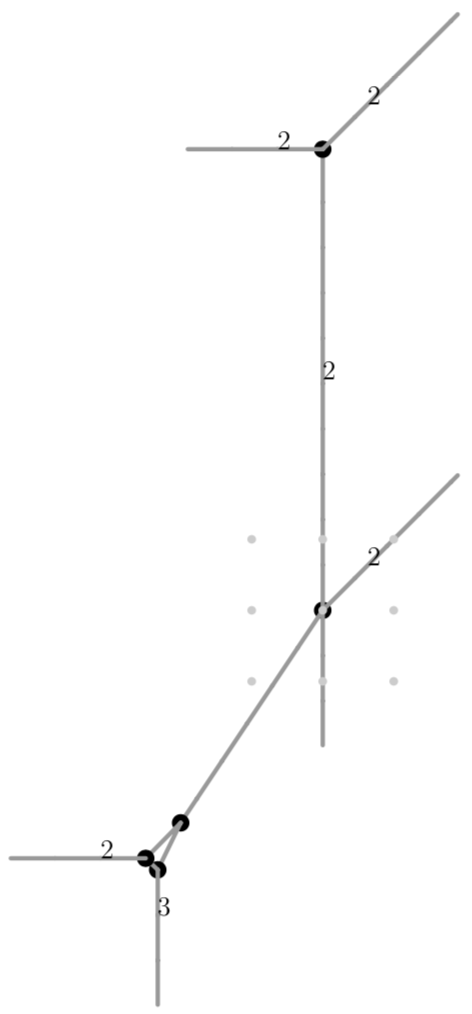# Type (303)¶

A tropical curve of type (303) is of the form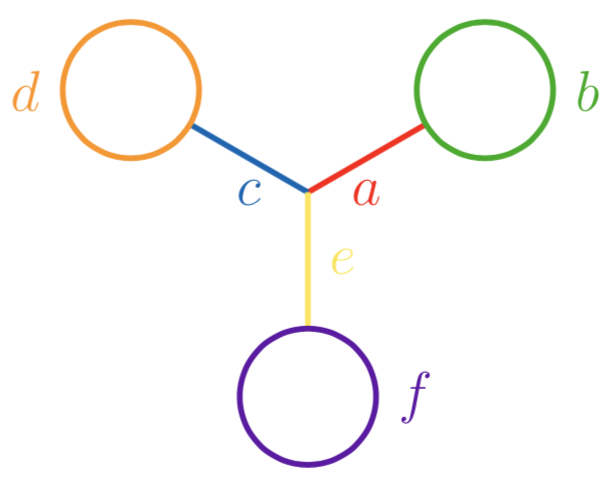Due to symmetry, we may assume $$a\geq c\geq e$$. It is never embeddable as a tropical quartic curve in $$\mathbb{R}^2$$.

To illustrate how a curve is embeddable in a tropical quartic curve as a tropical plane, consider the following configuration of lengths:

$a=1, \quad b=1, \quad c=1, \quad d=2, \quad e=1, \quad f=3.$

For that, consider the following quartic polynomial with its highly degenerate tropical quartic curve:

poly g = (t19)*x4+x2y2+(t13)*y4+(2t6+2t5+2t4+2t3+2t2+2t+2)*x2y+(2t4+2t3+2t2+2t+2)*xy2+(t12+2t11+3t10+4t9+5t8+6t7+6t6+6t5+5t4+4t3+3t2+2t+1)*x2+(-2t2-4t-2)*xy+(t8+2t7+3t6+4t5+4t4+4t3+3t2+2t+1)*y2+(t7);
drawTropicalCurve(g,"max");

$\begin{split}g &= t^{19}x^4+x^2y^2+t^{13}y^4+(2t^6+2t^5+2t^4+2t^3+2t^2+2t+2)x^2y\\ &\quad +(2t^4+2t^3+2t^2+2t+2)xy^2\\ &\quad +(t^{12}+2t^{11}+3t^{10}+4t^9+5t^8+6t^7+6t^6+6t^5+5t^4+4t^3+3t^2+2t+1)x^2\\ &\quad +(-2t^2-4t-2)xy+(t^8+2t^7+3t^6+4t^5+4t^4+4t^3+3t^2+2t+1)y^2+t^7\end{split}$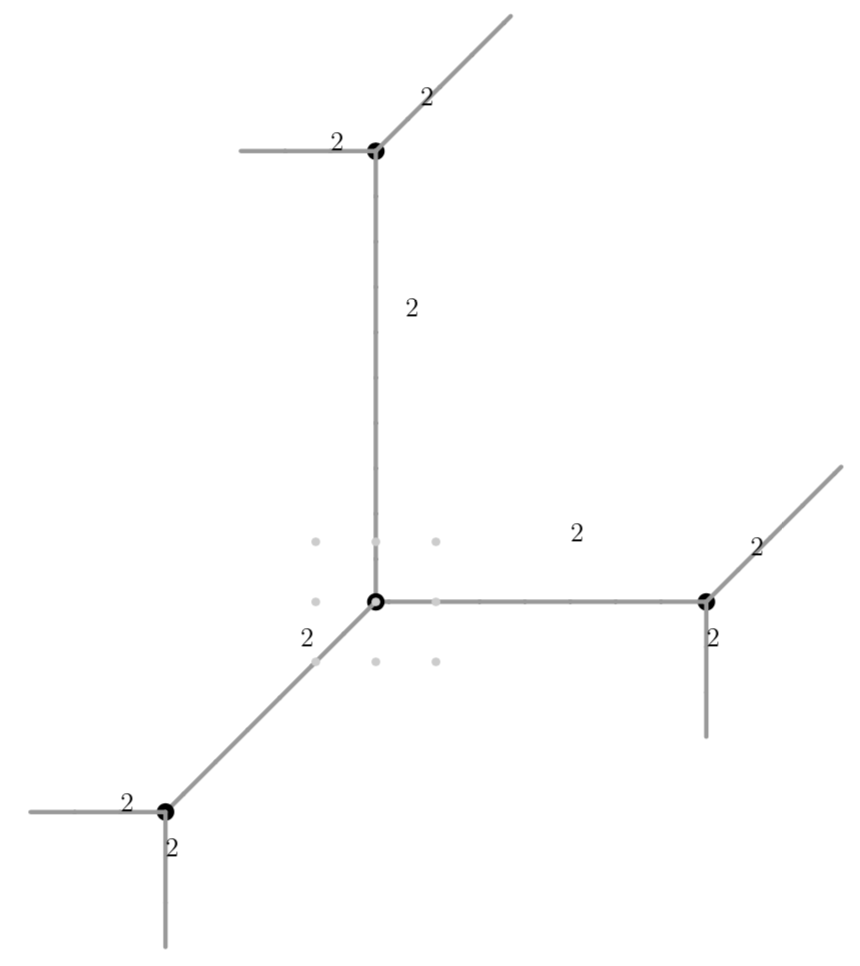The modification $$z_1=x+1+t+t^3+t^4$$, which goes along $$w_x=0$$, yields the following tropical curve after projecting onto the $$z_1y$$ plane ($$y$$ remaining the vertical direction):

poly g1=substitute(g,x,x-1-t-t3-t4);
drawTropicalCurve(g1,"max");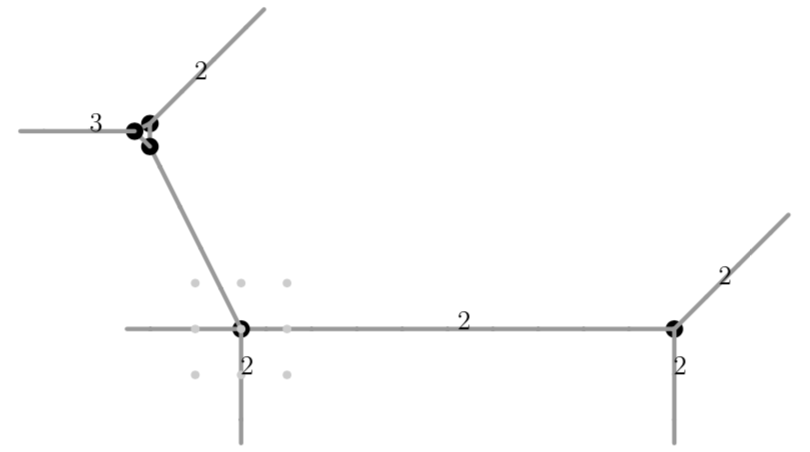The entirety of $$w_x<0$$ is mapped onto $$w_z=0$$, while the edge on the line $$w_x=0$$ with multiplicity $$2$$ reveals a lolli with edge $$e$$ of length $$1$$ and cycle $$b$$ of length $$1$$.

Similarly, the modifications $$z_2=y+1+t++t^2+t^4+t^5+t^6$$ and $$z_0=y-x-2itx$$, where $$i\in\mathbb C$$ denotes the imaginary number, will reveal lollis of desired edge and cycle lengths:

poly g2=substitute(g,y,y-1-t-t2-t4-t5-t6);
drawTropicalCurve(g2,"max");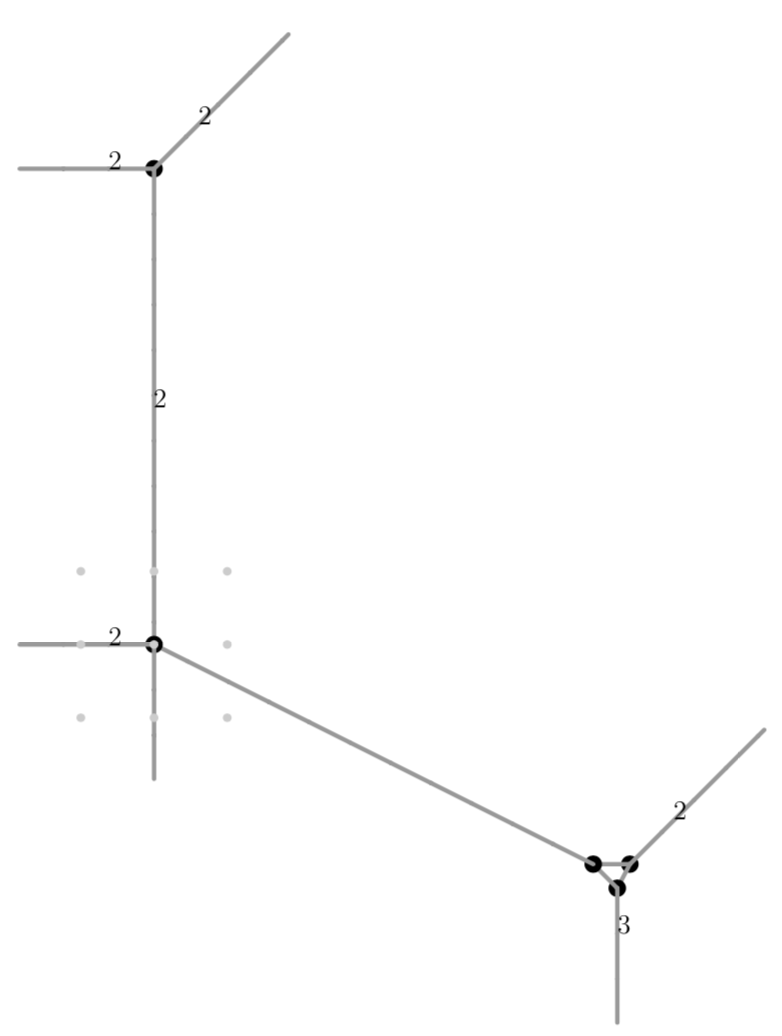ring s = (0,i),(t,x,y),dp;
minpoly = i2+1;
poly g = imap(r,g);
poly g0 = substitute(g,y,y+x+2it*x);
g0 = subst(g0,i,15);
setring r;
poly g0 = imap(s,g0);
drawTropicalCurve(g0,"max");


(Note that we are cheating slightly in the final modification, as we are setting $$i=15$$ when it comes to drawing the picture)